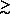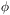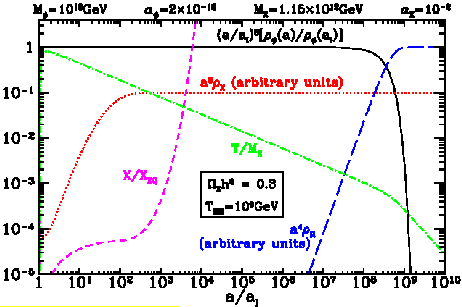5.2 WIMPZILLAS - Size Does Matter

The simple assumption that the dark matter (DM) is a thermal relic is surprisingly restrictive. The limitX1 implies that the mass of a DM relic must be less than about 500 TeV . The standard lore is that the hunt for DM should concentrate on particles with mass of the order of the weak scale and with interactions with ordinary matter on the scale of the weak force. This has been the driving force behind the vast effort in DM detectors.

But recent developments in understanding how matter is created in the early universe suggests the possibility that DM might be naturally composed of nonthermal supermassive states. The supermassive dark matter (WIMPZILLA) X may have a mass many orders of magnitude larger than the weak scale, possibly as large as the Grand Unified Theory (GUT) scale. It is very intriguing that these considerations resurrect the possibility that the dark matter might be charged or even strongly interacting!

The second condition for WIMPZILLAS is that the particle must not have been in equilibrium when it froze out (i.e., it is not a thermal relic), otherwiseX would be larger than one. A sufficient condition for nonequilibrium is that the annihilation rate (per particle) must be smaller than the expansion rate: n|v| < H, where n is the number density,|v| is the annihilation rate times the Møller flux factor, and H is the expansion rate. Conversely, if the WIMPZILLA was created at some temperature T* andX < 1, then it is easy to show that it could not have attained equilibrium. To see this, assume Xs were created in a radiation-dominated universe at temperature T*. ThenX is given byX =(T*/T0) MX nX(T*) /(T*), where T0 is the present temperature (ignoring dimensionless factors of order unity.) Using the fact that(T*) = H(T*) MPl T*2, we find nX(T*) / H(T*) = (X /) T0 MPl T* / MX. We may safely take the limit|v| < MX-2, so nX(T*)|v| / H(T*) must be less than (X /) T0 MPl T* / MX3. Thus, the requirement for nonequilibrium is(14)

This implies that if a nonrelativistic particle with MX200 TeV was created at T* < MX with a density low enough to result inX1, then its abundance must have been so small that it never attained equilibrium. Therefore, if there is some way to create WIMPZILLAS in the correct abundance to giveX ~ 1, nonequilibrium is guaranteed.

An attractive origin for WIMPZILLAS is during the defrosting phase after inflation. It is important to realize that it is not necessary to convert a significant fraction of the available energy into massive particles; in fact, it must be an infinitesimal amount. If a fractionof the available energy density is in the form of a massive, stable X particle, thenX =(TRH / T0), where TRH is the ``reheat'' temperature. ForX = 1, this leads to the limit10-17 (109 GeV / TRH).

In one extreme we might assume that the vacuum energy of inflation is immediately converted to radiation, resulting in a reheat temperature TRH. In this caseX can be calculated by integrating the Boltzmann equation with initial condition NX = 0 at T = TRH. One expects the X density to be suppressed by exp(-2MX / TRH); indeed, one findsX ~ 1 for MX / TRH ~ 25 + 0.5 ln(MX2 <|v| >), in agreement with previous estimates  that for TRH ~ 109 GeV, the WIMPZILLA mass would be about 2.5 x 1010 GeV.

A second (and more plausible) scenario is that reheating is not instantaneous, but is the result of the decay of the inflaton field. In this approach the radiation is produced as the inflaton decays. The WIMPZILLA density is found by solving the coupled system of equations for the inflaton field energy, the radiation density, and the WIMPZILLA mass density. The calculation has been recently reported in Ref. , with resultX ~ MX2 <|v| > (2000 TRH / MX)7. For a reheat temperature as low as 109 GeV, a particle of mass 1013 GeV can be produced in sufficient abundance to giveX ~ 1.

The large difference in WIMPZILLA masses in the two reheating scenarios arises because the peak temperature is much larger in the second scenario, even with identical TRH. Because the temperature decreases as a-3/8 (a is the scale factor) during most of the reheating period in the second scenario, it must have once been much greater than TRH. The evolution of the temperature is given in Fig. 9. If we assume the radiation spectrum did not depart grossly from thermal, the effective temperature having once been larger than TRH implies that the density of particles with enough energy to create WIMPZILLAS was larger. Denoting as T2 the maximum effective temperature for the second scenario, we find T2 / TRH ~ (M/)1/4 >> 1, whereis the effective decay rate of the inflaton. See  for details.Figure 9. The evolution of energy densities and T/MX as a function of the scale factor. Also shown is X/XEQ.

Another way to produce WIMPZILLAS after inflation is in a preliminary stage of reheating called ``preheating'' , where nonlinear quantum effects may lead to an extremely effective dissipational dynamics and explosive particle production. Particles can be created in a broad parametric resonance with a fraction of the energy stored in the form of coherent inflaton oscillations at the end of inflation released after only a dozen oscillation periods. A crucial observation for our discussion is that particles with mass up to 1015 GeV may be created during preheating [10, 11, 12], and that their distribution is nonthermal. If these particles are stable, they may be good candidates for WIMPZILLAS.

To study how the creation of WIMPZILLAS takes place in preheating, let us take the simplest chaotic inflation potential: V () = M22 / 2 with M~ 1013 GeV. We assume that the interaction term between the WIMPZILLA and the inflaton field is of the type g22 |X|2. Quantum fluctuations of the X field with momentumduring preheating approximately obey the Mathieu equation, X''k + [A (k) - 2q cos2z] Xk = 0, where q = g22 / 4 M2, A (k) = (k2 + MX2) / M2 + 2q (primes denotes differentiation with respect to z = Mt). Particle production occurs above the line A = 2q in an instability strip of width scaling as q1/2 for large q. The condition for broad resonance, A - 2qq1/2 , becomes (k2 + M2X) / M2g bar/ M, which yields EX2 = k2 + MX2g barMfor the typical energy of particles produced in preheating. Here baris the amplitude of the oscillating inflaton field . The resulting estimate for the typical energy of particles at the end of the broad resonance regime for M~ 10-6 MPl is EX ~ 10-1 g1/2 sqrt (MMPl) ~ g1/2 1015 GeV. Supermassive X bosons can be produced by the broad parametric resonance for EX > MX, which leads to the estimate that X production will be possible if MX < g1/2 1015 GeV. For g2 ~ 1 one would have copious production of X particles as heavy as 1015 GeV, i.e., 100 times greater than the inflaton mass, which may be many orders of magnitude greater than the reheat temperature. Scatterings of X fluctuations off the zero mode of the inflaton field considerably limits the maximum magnitude of X fluctuations to be < X2 >maxM2 / g2 . For example, < X2 >max10-10 MPl2 in the case MX = 10 M. This restricts the corresponding number density of created X-particles.

For a reheating temperature of the order of 100 GeV, the present abundance of WIMPZILLAS with mass MX ~ 1014 GeV is given byX ~ 1 if~ 10-10. This small fraction corresponds to < X2 > ~ 10-12 MPl2 at the end of the preheating stage, a value naturally achieved for WIMPZILLA mass in the GUT range . The creation of WIMPZILLAS through preheating and, therefore, the prediction of the present value ofX, is very model dependent. The inflaton might preferably decay through parametric resonance into very light boson fields so that the end of the preheating stage and of the corresponding value of < X2 > depends upon the coupling of the inflaton field not only to the WIMPZILLA, but also to other degrees of freedom. It is encouraging, however, that it is possible to produce supermassive particles during preheating that are as massive as 1012 TRH. Details of WIMPZILLA production in preheating can be found in .

Another possibility which has been recently investigated is the production of very massive particles by gravitational mechanisms [15, 16]. In particular, the desired abundance of WIMPZILLAS may be generated during the transition from the inflationary phase to a matter/radiation dominated phase as the result of the expansion of the background spacetime acting on vacuum quantum fluctuations of the dark matter field . A crucial side-effect of the inflationary scenarios is the generation of density perturbations. A related effect, which does not seem to have attracted much attention, is the possibility of producing matter fields due to the rapid change in the evolution of the scale factor around the end of inflation. Contrary to the first effect, the second one contributes to the homogeneous background energy density that drives the cosmic expansion, and is essentially the familiar ``particle production'' effect of relativistic field theory in external fields.

Very massive particles may be created in a nonthermal state with sufficient abundance to achieve critical density today by the classical gravitational effect on the vacuum state at the end of inflation. Mechanically, the particle creation scenario is similar to the inflationary generation of gravitational perturbations that seed the formation of large-scale structures. However, the quantum generation of energy density fluctuations from inflation is associated with the inflaton field, which dominated the mass density of the universe, and not a generic sub-dominant scalar field.

If 0.04MX / He2 , where He is the Hubble constant at the end of inflation, DM produced gravitationally can have a density today of the order of the critical density. This result is quite robust with respect to the fine details of the transition between the inflationary phase and the matter-dominated phase. The only requirement is that(15)

The observation of anisotropy in the cosmic background radiation does not fix He uniquely, but using TRHsqrt (MPl He), we find that the mechanism is effective only when He109 GeV (or, MX108 GeV).

The distinguishing feature of this mechanism  is the capability of generating particles with mass of the order of the inflaton mass even when the WIMPZILLA interacts only extremely weakly (or not at all!) with other particles, including the inflaton. This feature makes the gravitational production mechanism quite model independent and, therefore, more appealing to us than the one occurring at preheating.

WIMPZILLAS can also be produced in theories where inflation is completed by a first-order phase transition . In these scenarios, the universe decays from its false vacuum state by bubble nucleation . When bubbles form, the energy of the false vacuum is entirely transformed into potential energy in the bubble walls, but as the bubbles expand, more and more of their energy becomes kinetic and the walls become highly relativistic. Eventually the bubble walls collide.

During collisions, the walls oscillate through each other  and the kinetic energy is dispersed into low-energy scalar waves [19, 20]. If these soft scalar quanta carry quantum numbers associated with some spontaneously broken symmetry, they may even lead to the phenomenon of nonthermal symmetry restoration . We are, however, more interested in the fate of the potential energy of the walls, MP = 4R2, whereis the energy per unit area of the bubble with radius R. The bubble walls can be imagined as a coherent state of inflaton particles, so that the typical energy E of the products of their decays is simply the inverse thickness of the wall, E ~-1. If the bubble walls are highly relativistic when they collide, there is the possibility of quantum production of nonthermal particles with mass well above the mass of the inflaton field, up to energy-1 =M,being the relativistic Lorentz factor.

Suppose now that the WIMPZILLA is some fermionic degree of freedom X and that it couples to the inflaton field by the Yukawa coupling gbarX X. One can treat(the bubbles or walls) as a classical, external field and the WIMPZILLA as a quantum field in the presence of this source. This amounts to ignoring the backreaction of particle production on the evolution of the walls, but this is certainly a good approximation in our case. The number of WIMPZILLA particles created in the collisions from the wall's potential energy is NX ~ fX MP / MX, where fX parametrizes the fraction of the primary decay products that are WIMPZILLAS. The fraction fX will depend in general on the masses and the couplings of a particular theory in question. For the Yukawa coupling g, it is fXg2 ln(M/ 2 MX) [20, 22]. Supermassive particles in bubble collisions are produced out of equilibrium and they never attain chemical equilibrium. Assuming TRH100 GeV, the present abundance of WIMPZILLAS isX ~ 1 if g ~ 10-51/2. Here-1 << 1 denotes the fraction of the bubble energy at nucleation which has remained in the form of potential energy at the time of collision. This simple analysis indicates that the correct magnitude for the abundance of X particles may be naturally obtained in the process of reheating in theories where inflation is terminated by bubble nucleation.

In conclusion, a large fraction of the DM in the universe may be made of WIMPZILLAS of mass greatly exceed the electroweak scale - perhaps as large as the GUT scale. This is made possible by the fact that the WIMPZILLAS were created in a nonthermal state and never reached chemical equilibrium with the primordial plasma.

ACKNOWLEDGEMENTS

This work was supported in part by the Department of Energy, as well as NASA under grant number NAG5-7092. The hospitality of Tom Ferbel and the inquisitiveness of the students were greatly appreciated.